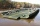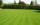# Bag of peanuts

Joe eat 1/3 of a bag of peanuts, mark eat 1/4 of the remaining in the bag of peanuts, Alvin eat 1/2 of the remaining bag of peanuts, peter eat 10 peanuts, there are 71 peanuts left. Hon many peanuts were in the bags?

Result

n =  324

#### Solution:

n/3 + 1/4 * (n-n/3) + 1/2*(n-(n/3 + 1/4 * (n-n/3))) + 10 + 71 = n

3n = 972

n = 324

Calculated by our simple equation calculator.

Leave us a comment of this math problem and its solution (i.e. if it is still somewhat unclear...):Be the first to comment!#### Following knowledge from mathematics are needed to solve this word math problem:

Need help calculate sum, simplify or multiply fractions? Try our fraction calculator. Do you have a linear equation or system of equations and looking for its solution? Or do you have quadratic equation?

## Next similar math problems:

1. Cleaning windowsCleaning company has to wash all the windows of the school. The first day washes one-sixth of the windows of the school, the next day three more windows than the first day and the remaining 18 windows washes on the third day. Calculate how many windows ha
2. PearsThere were pears in the basket, I took two-fifths of them, and left six in the basket. How many pears did I take?
3. Simple equationSolve for x: 3(x + 2) = x - 18
4. Unknown numberI think the number - its sixth is 3 smaller than its third.
5. One thirdIf 3/5 is 360, how much is 1/3?
6. UN 1If we add to an unknown number his quarter, we get 210. Identify unknown number.
7. FractionsThree-quarters of an unknown number are 4/5. What is 5/6 of this unknown number?
8. Fraction + eqSolve following simple equation with fractions: -5/6(8+5b) = 75 + 5/3b
9. EquationSolve the equation: 1/2-2/8 = 1/10; Write the result as a decimal number.
10. New bridgeThanks to the new bridge, the road between A and B has been cut to one third and is now 10km long. How much did the road between A and B measure before?
11. Simple equation 6Solve equation with one variable: X/2+X/3+X/4=X+4
12. Unknown numberIdentify unknown number which 1/5 is 40 greater than one tenth of that number.
13. EqnSolve equation with fractions: 2x/3-50=40+x/4
14. Eq-fracSolve the following equation with fractions: h + 1/3 =5/3
15. Equation with fractionsSolve equation: ? It is equation with fractions.
16. Equation with xSolve the following equation: 2x- (8x + 1) - (x + 2) / 5 = 9
17. Mr. HappyMr. Happy planted 36.6 meters square gardens grass; It's a third of the garden more than half of the garden. What is square area of this garden?